﻿ Dialog: Function-Block: Graph

# Dialog: Function-Block: Graph

## Graph FB

### Graph FB

Use a Graph FB to plot Kinematic Data, Kinetostatic Force-Data and Cam-Data.

Y-axis input-connectors: The top four input-connectors are for the Y-axis of each graph.

X-axis input-connector:  The bottom input-connector scales the X-axis. If you do not connect a wire to this connector, the plots are made over one machine cycle, 0 –360.

You can connect any wire with motion-values as the data-type to the bottom input-connector. I nearly always connector a Linear-Motion FB to the X-axis input-connector.

### Edit Graph FBToolbar:

Kinematic FB toolbar : Add Graph FB

 1 Click Kinematics FB-Block Toolbar > Graph FB
 2 Click again in the graphic-area to 'place' the Graph FB in the graphic-area and the Assembly-Tree

The Graph FB is now in the graphic-area.

Edit:

To open the Graph dialog-box:

 1 Double-click a Graph FB icon in the graphic-area or the Assembly-Tree

or

 1

### Graph FB dialog-box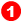Y–axis Scales:

 • 'Y-Scale' for the data with each input – from left to right, Y1 to Y4 [Y1 is the top input-connector to the Graph FB].
 • The colour of the Y–axis Scale and the graph-line are the same.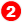Y–axis data-Channel [Drop-Down list]

 • Each wire that is connected to an input-connector has up to 3 Data-Channels.
 • You can select to plot which of the three different Data-Channels.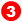Digital Readout (DRO):

 • The X–axis and the Y1 to Y4-axis values at the position of the vertical cursor.
 • The Number-Format for the X–axis, Y–axis, and 'Data in Clipboard', is configured in Application Settings | Number Format tab.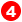The Graph Area:

 • The colour-coded graphs for each Y–axis input
 • Vertical-CursorThe 'Vertical Red Pointer' AND the Master Machine Angle 'jump' to where you click in the graph area, and also to where you 'Drag' your mouse pointer in the graph.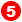Toolbar:

 • Save, Print, Graph Settings, Zoom Extents, Pan Left, Pan Right, 'Copy Graph Data to Clipboard' – seebelow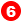Vertical Cursor:

When you click in the Graph Area, the Vertical Cursor will snap to your mouse Pointer.

When you drag your pointer in the graphic-area:

 • the Vertical Cursor will move with your Pointer
 • the Digital Readoutswill continuously update to be the same as the data values at the position of the pointer along the X–axis
 • the Master Machine Angle will continuously update to be the same as the position of the Vertical Red Pointer along the X–axis
 • the kinematically-defined Chains will move to agree with the Master Machine Angle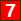Copy Graph Data to Clipboard

 • When you click the right-most tool/icon in the toolbarabove the Graphs, the graph data is copied to the Clipboard.

Paste this Data to Excel, or to MotionDesigner in the Data-Transfer table, where you can plot it as an overlay.

You do not need to copy the data to the clipboard - simply Paste the data immediately after the data shows in the table.

If necessary, change the Number-Format for the data in Application Settings | Number Format tab.

Why are the Y–axis values given by the DROslightly different to values of Vectors I can see in the graphic-area?

The values may be slightly different because, in the graph, the X–axis and Y–axis values at the DRO must jump from 'machine -step' to 'machine-step', with each step found by the Number-of-Steps' parameter in the Machine Settings dialog-box.

In the graphic area, the vector values are calculated for the value of the Master Machine Angle, which may not coincide with a 'machine step'.

#### Y-Axis Data-Channels :

The 'Y-Axis Data Channels Set' are given in the drop-down at the right of the graph dialog-box.

If you want to show more than one of the data options for one of the FBs listed below, then you must drag a wire from the same output-connector to different input-connectors on the Graph FB.

You can plot:

 o Three Data-Types available at the output-connector of a FB
 o To trouble-shoot. For example, the motion given to a Rocker or Slider is not as you expect.
 o To learn how FBs transform the motion data.
 • Function-Block (Linear-Motion, Gearing, Motion, Motion-Dimension) you can plot:
 o Linear or angular position
 o Linear or angular velocity
 o Linear or angular acceleration
 • Measurement FB or Point-Data FB you can plot
 o Linear or angular position
 o Linear or angular velocity
 o Linear or angular acceleration
 • Cam-Data FB
 o Pressure Angle. You can plot three Pressure Angles – See Pressure Angle
 o Cam Radius-of-Curvature. You can plot Radii-of-Curvature 1 and 2 for Cam 1 and Cam 2.
 o Contact Cam Force
 o Maximum Contact Shear Stress. You must know the material properties to obtain this data. See Cam Properties and Display dialog-box.
 • Force-Data FB

The output form a Force-Data FB is a function of the element with which it is linked.

If you link a Force-Data FB to a:

 o Pin-Joint with a Motor, you can plot Motor Drive Torque.
 o Slide-Joint with a Motor, you can plot Motor Drive Linear Force
 o Joint without a Motor, plot
 ▪ Total Force [ FT = √(FX2 + FY2), or
 ▪ Force along the X-coordinate of a Point: FX
 ▪ Force along the Y-coordinate of a Point: FY
 o 2D-Cam, you can plot the Cam Contact Force
 o Spring FB: you can plot the Total Force, the X Force and the Y Force acting on the Point.

Tutorial and Reference Help Files for MechDesigner and MotionDesigner 13.2 + © Machine, Mechanism, Motion and Cam Design Software by PSMotion Ltd